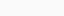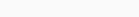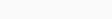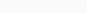# Coursera machine learning week 2 Quiz answer Linear Regression with Multiple Variables | Andrew NG

In this article, you will find Coursera machine learning week 2 Quiz answer Linear Regression with Multiple Variables | Andrew Ng. Use “Ctrl+F” To Find Any Questions or Answers. For Mobile Users, You Just Need To Click On Three dots In Your Browser & You Will Get A “Find” Option There. Use These Options to Get Any Random Questions Answer.

Try to solve all the assignments by yourself first, but if you get stuck somewhere then feel free to browse the code. Don’t just copy-paste the code for the sake of completion.  Even if you copy the code, make sure you understand the code first.

### Coursera machine learning week 2 Quiz answer Linear Regression with Multiple Variables

1. Suppose m=4 students have taken some classes, and the class had a midterm exam and a final exam. You have collected a dataset of their scores on the two exams, which is as follows:You’d like to use polynomial regression to predict a student’s final exam score from their midterm exam score. Concretely, suppose you want to fit a model of the form , whereis the midterm score and x_2 is (midterm score)^2. Further, you plan to use both feature scaling (dividing by the “max-min”, or range, of a feature) and mean normalization.
What is the normalized feature  ? (Hint: midterm = 69, final = 78 is training example 4.) Please round off your answer to two decimal places and enter in the text box below.
2. You run gradient descent for 15 iterations withand compute after each iteration. You find that the value ofdecreases slowly and is still decreasing after 15 iterations. Based on this, which of the following conclusions seems most plausible?

2. You run gradient descent for 15 iterations withand compute after each iteration. You find that the value ofdecreases quickly then levels off. Based on this, which of the following conclusions seems most plausible?

3. Suppose you have m = 23 training examples with n = 5 features (excluding the additional all-ones feature for the intercept term, which you should add). The normal equation is . For the given values of m and n, what are the dimensions of, X, and y in this equation?

•  X is 23 × 5, y is 23 × 1, θ is 5 × 5
•  X is 23 × 6, y is 23 × 6, θ is 6 × 6
•  X is 23 × 6, y is 23 × 1, θ is 6 × 1
•  X is 23 × 5, y is 23 × 1, θ is 5 × 1

4. Suppose you have a dataset with m = 1000000 examples and n = 200000 features for each example. You want to use multivariate linear regression to fit the parametersto our data. Should you prefer gradient descent or the normal equation?

•  Gradient descent, since it will always converge to the optimal θ.
•  Gradient descent, since  will be very slow to compute in the normal equation.

With n = 200000 features, you will have to invert a 200001 x 200001 matrix to compute the normal equation. Inverting such a large matrix is computationally expensive, so gradient descent is a good choice.

• The normal equation, since it provides an efficient way to directly find the solution.
•  The normal equation, since gradient descent might be unable to find the optimal θ.

5. Which of the following are reasons for using feature scaling?

•  It is necessary to prevent gradient descent from getting stuck in local optima.
•  It speeds up solving for θ using the normal equation.

The magnitute of the feature values are nsignificant in terms of computational cost.

Feature scaling has nothing to do with matrix inversion.

•  It speeds up gradient descent by making it require fewer iterations to get to a good solution.

Feature scaling speeds up gradient descent by avoiding many extra iterations that are required when one or more features takes on much larger values than he rest.

Disclaimer:  Hopefully, this article will be useful for you to find all the Coursera machine learning week 2 Quiz answer Linear Regression with Multiple Variables– Andrew NG and grab some premium knowledge with less effort.
Finally, we are now, in the end, I just want to conclude some important message for you, Feel free to ask doubts in the comment section. I will try my best to answer it. If you find this helpful by any means like, comment, and share the post. Please share our posts on social media platforms and also suggest to your friends to Join Our Groups. Don’t forget to subscribe. This is the simplest way to encourage me to keep doing such work.
Is Andrew Ng’s Machine Learning course good?
It is the Best Course for Supervised Machine Learning! Andrew Ng Sir has been like always has such important & difficult concepts of Supervised ML with such ease and great examples, Just amazing!
How do I get answers to coursera assignment?
Use “Ctrl+F” To Find Any Questions Answered. & For Mobile Users, You Just Need To Click On Three dots In Your Browser & You Will Get A “Find” Option There. Use These Options to Get Any Random Questions Answer.
How long does it take to finish coursera Machine Learning?
this specialization requires approximately 3 months with 75 hours of materials to complete, and I finished it in 3 weeks and spent an additional 1 week reviewing the whole course.
How do you submit assignments on Coursera Machine Learning?
Submit a programming assignment Open the assignment page for the assignment you want to submit. Read the assignment instructions and download any starter files. Finish the coding tasks in your local coding environment. Check the starter files and instructions when you need to. Reference

Sharing Is Caring